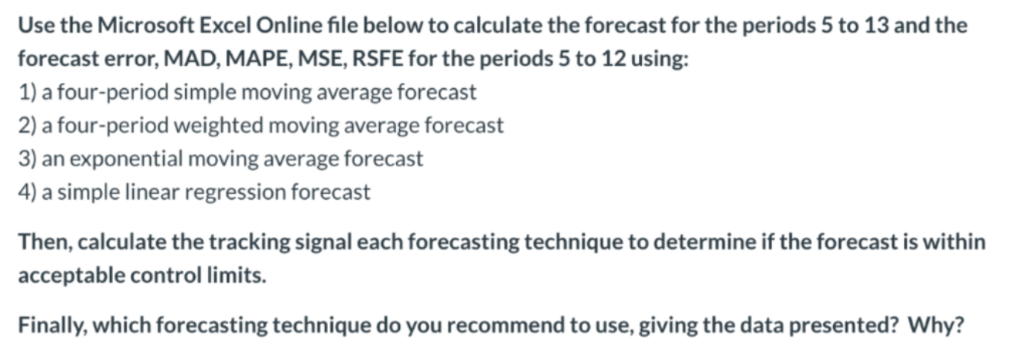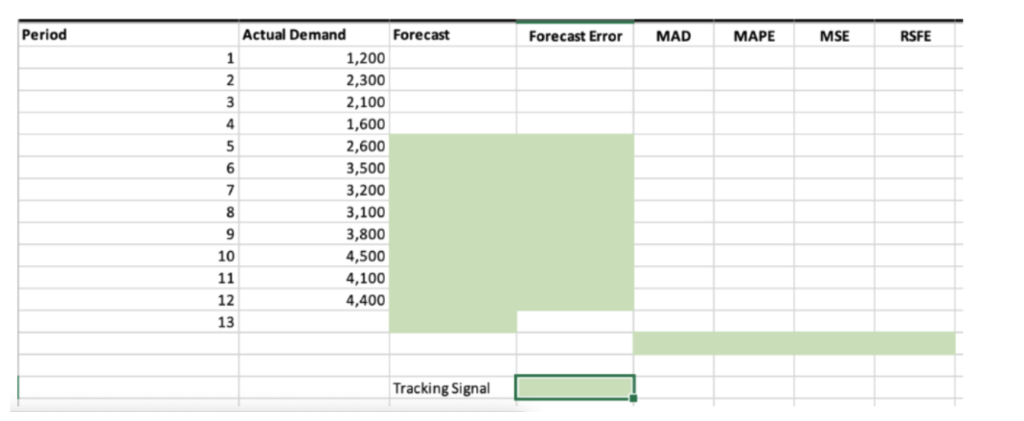# Solved: Use The Microsoft Excel Online File Below To Calculate The Forecast For The Periods 5 To 13 And The Forecast Error, MAD, MAPE, MSE, RSFE For The Periods 5 To 12 Using 1) A Four-period Simple M

By |Use the Microsoft Excel Online file below to calculate the forecast for the periods 5 to 13 and the forecast error, MAD, MAPE, MSE, RSFE for the periods 5 to 12 using 1) a four-period simple moving average forecast 2) a four-period weighted moving average forecast exponential moving average forecast 4) a simple linear regression forecast 3) an Then, calculate the tracking signal each forecasting technique to determine if the forecast is within acceptable control limits. Finally, which forecasting technique do you recommend to use, giving the data presented? Why? Period Actual Demand Forecast Forecast Error MAPE MSE RSFE MAD 1,200 1 2 2,300 3 2,100 4 1,600 5 2,600 6 3,500 7 3,200 3,100 3,800 4,500 10 11 4,100 12 4,400 13 Tracking Signal